Question

# A piece of soap of mass m, initially at rest, is released onto a slippery track...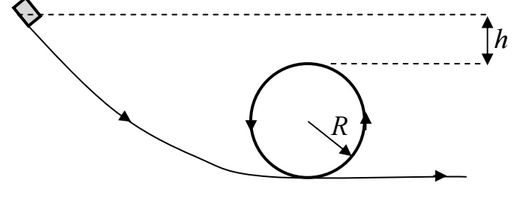A piece of soap of mass m, initially at rest, is released onto a slippery track from a height h = R/2 above the top of a loop of radius R (see diagram below). What is the magnitude of the normal force acting on the soap as it begins its circular trajectory at the base of the loop?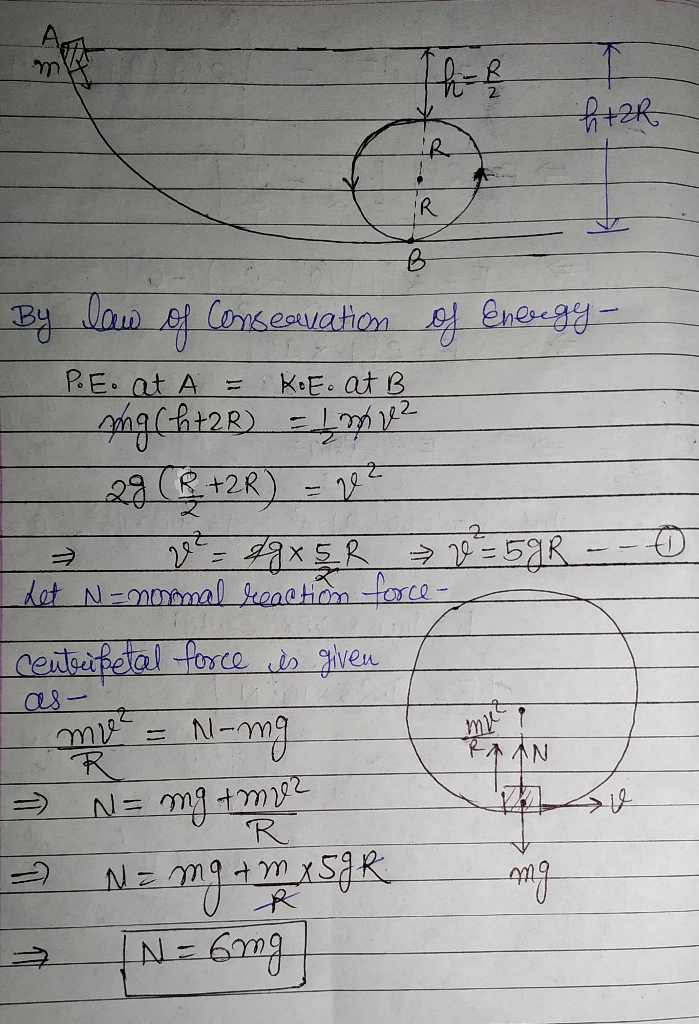#### Earn Coins

Coins can be redeemed for fabulous gifts.

Similar Homework Help Questions
• ### A 48.5-g toy car is released from rest on a frictionless track with a vertical loop...

A 48.5-g toy car is released from rest on a frictionless track with a vertical loop of radius R. The initial height of the car is h = 4.45R. (a) What is the speed of the car at the top of the vertical loop? (Use the following as necessary: R and g.) (b) What is the magnitude of the normal force acting on the car at the top of the vertical loop?

• ### A 58.0-g toy car is released from rest on a frictionless track with a vertical loop...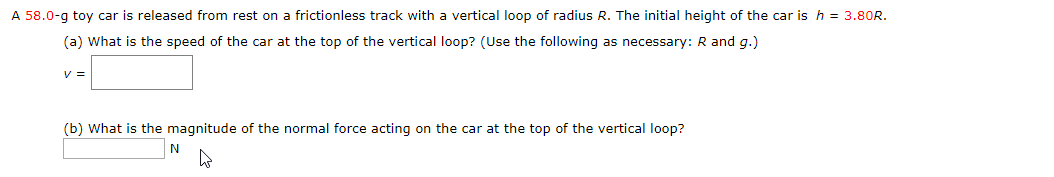A 58.0-g toy car is released from rest on a frictionless track with a vertical loop of radius R. The initial height of the car is h 3.80R. (a) What is the speed of the car at the top of the vertical loop? (Use the following as necessary: R and g.) v= (b) What is the magnitude of the normal force acting on the car at the top of the vertical loop?

• ### A roller coaster car of mass 800 kg when released from rest at point A (height...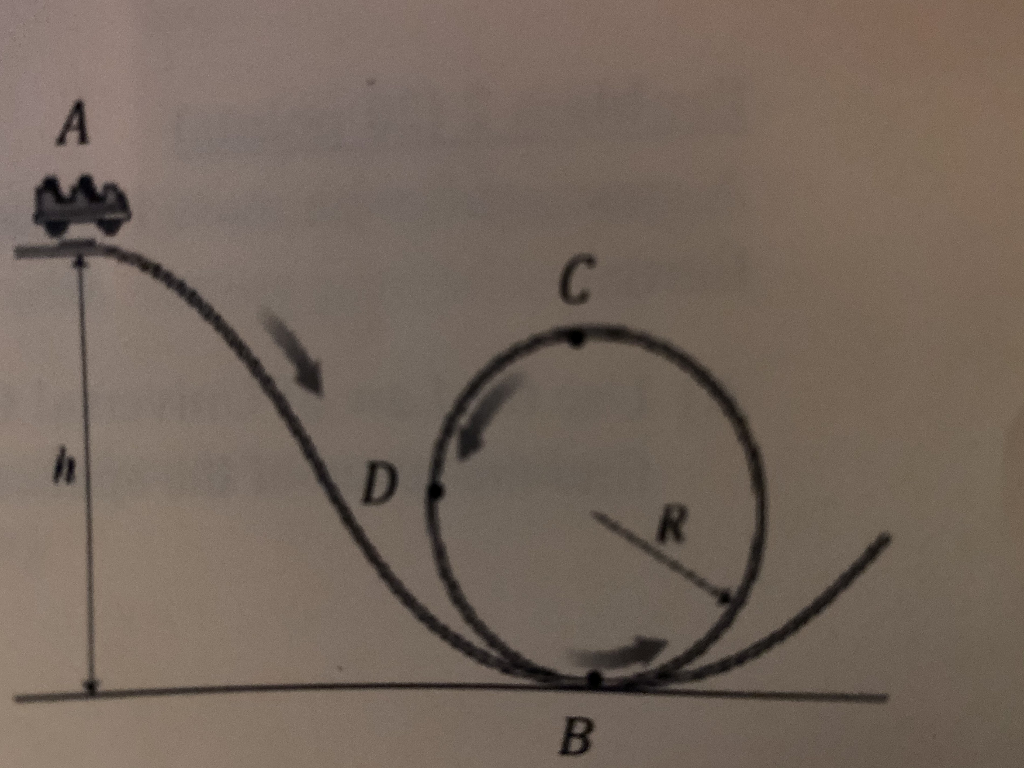A roller coaster car of mass 800 kg when released from rest at point A (height h above the ground) slides along the track and inside the loop of radius 16.0 m. The car never loses contact with the track. A) Draw below a free body diagram for the car the car at the top of the loop. B) If the normal force acting on the car at the top is 1500 N, what is the speed of the car...

• ### A roller coaster car of mass 800 kg when released from rest at point A (height...A roller coaster car of mass 800 kg when released from rest at point A (height h above the ground) slides along the track and inside the loop of radius 16.0 m. The car never loses contact with the track. A) Draw below a free body diagram for the car the car at the top of the loop. B) If the normal force acting on the car at the top is 1500 N, what is the speed of the car...

• ### In the figure here, a solid brass ball of mass 0.298 g will roll smoothly along...In the figure here, a solid brass ball of mass 0.298 g will roll smoothly along a loop-the-loop track when released from rest along the straight section. The circular loop has radius R = 0.19 m, and the ball has radius r << R. (a) What is h if the ball is on the verge of leaving the track when it reaches the top of the loop? (b) If the ball is released at height h = 7R, what is...

• ### A small block of mass m slides along the frictionless loop the loop track shown below....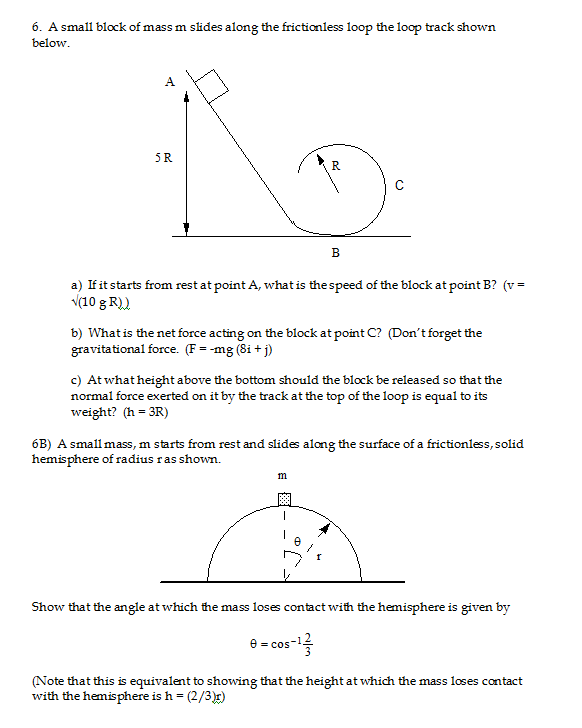A small block of mass m slides along the frictionless loop the loop track shown below. If it starts from rest at point A, what is the speed of the block at point B? (v = squareroot (10 g R)) What is the net force acting on the block at point C? (Don't forget the gravitational force. (F = -mg (8i + j) At what height above the bottom should the block be released so that the normal force exerted...

• ### In the figure, a small block of mass m = 0.022 kg can slide along the...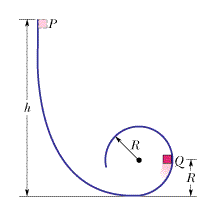In the figure, a small block of mass m = 0.022 kg can slide along the frictionless loop-the-loop, with loop radius R = 15 cm. The block is released from rest at point P, at height h = 5R above the bottom of the loop. What are the magnitudes of (a) the horizontal component and (b) the vertical component of the net force acting on the block at point Q? (c) At what height h should the block be released...

• ### Consider a solid sphere of mass m and radius r being released from a height h...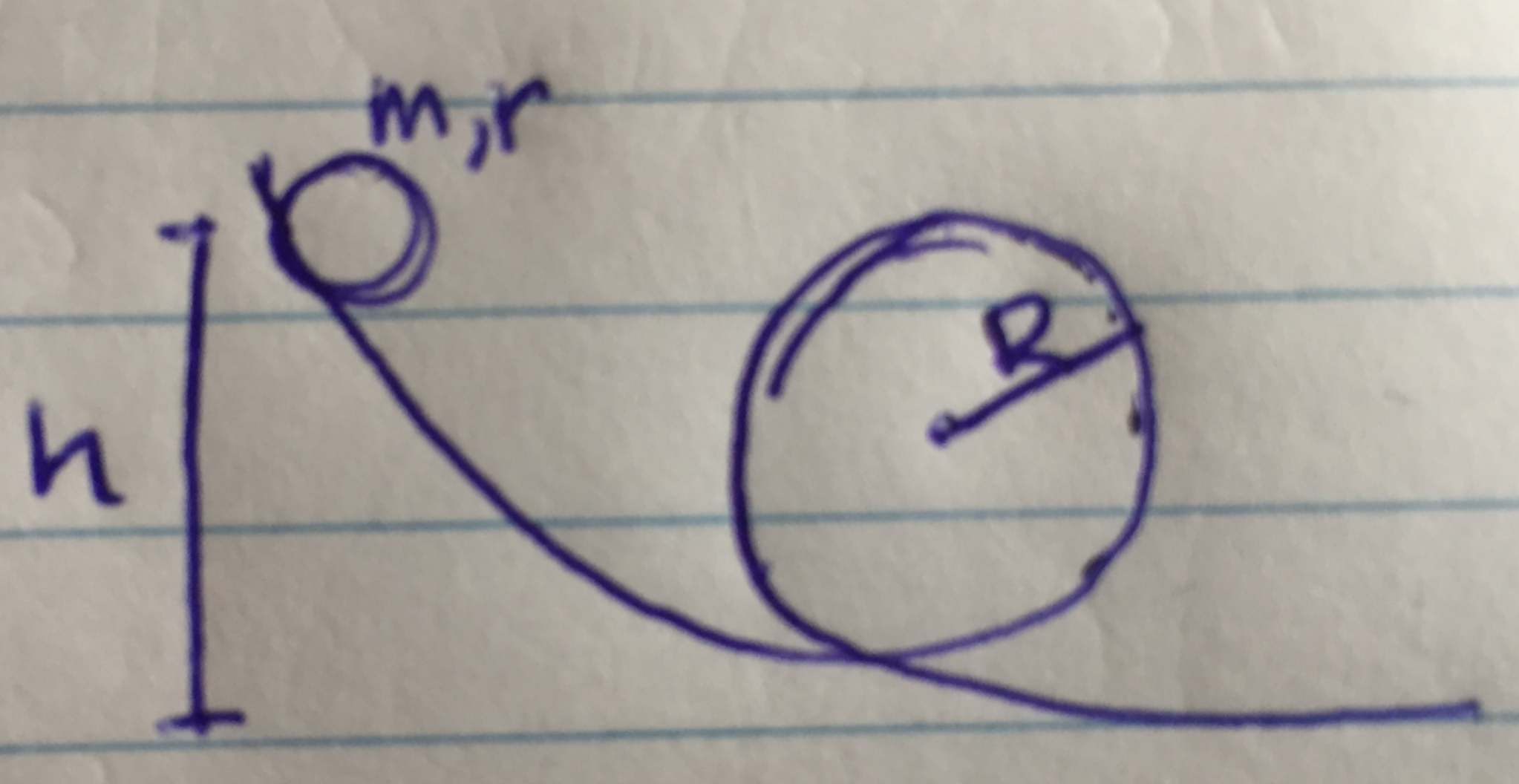Consider a solid sphere of mass m and radius r being released from a height h (i.e., its center of mass is initially a height h above the ground). It rolls without slipping and passes through a vertical loop of radius R. a. Use energy conservation to determine the tangential and angular velocities of the sphere when it reaches the top of the loop. b. Draw a force diagram for the sphere at the top of the loop and write...

• ### AP Physics C FRQ 3. A sphere of mass m and radius r is released from...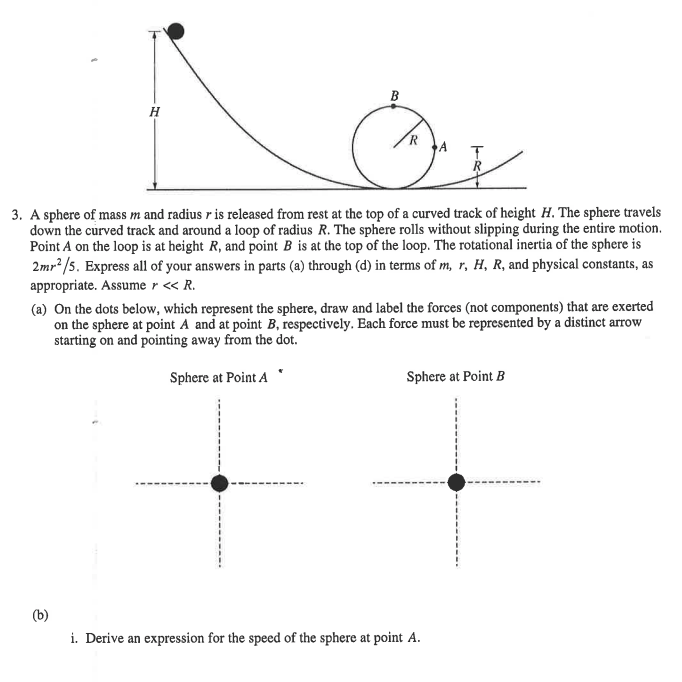AP Physics C FRQ 3. A sphere of mass m and radius r is released from rest at the top of a curved track of height H. The sphere travels down the curved track and around a loop of radius R. The sphere rolls without slipping during the entire motion. Point A on the loop is at height R, and point B is at the top of the loop. The rotational inertia of the sphere is 2mr2/s. Express all of...

• ### Problem 9 m,r A solid ball of mass m and radius r sits at rest at the top of a hill of height H l...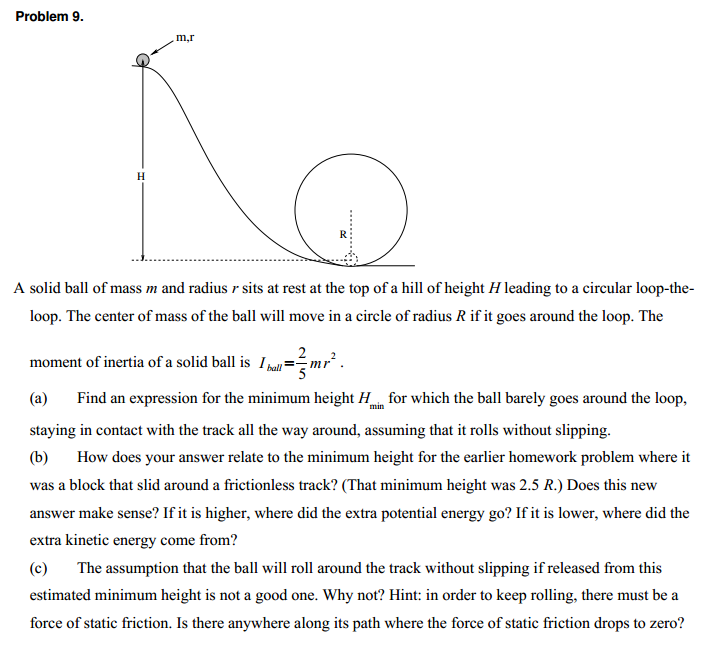Problem 9 m,r A solid ball of mass m and radius r sits at rest at the top of a hill of height H leading to a circular loop-the loop. The center of mass of the ball will move in a circle of radius R if it goes around the loop. The moment of inertia of a solid ball is Ibull--mr. (a) Find an expression for the minimum height H for which the ball barely goes around the loop, staying...